# The role of the betweenness centrality measure in networks

Ever wondered how to detect the most influential individual, station, motorway or node in a network? It is not a normal popularity test but a mathematical way for determining a node with the most impact in the flow of information within a network. A very good way of determining nodes that are great connectors for moving from one point of a graph to another. In a real-world situation, when these nodes are removed, the movement to other nodes in the graph becomes quite challenging. With betweenness centrality, the number of paths a node is a part of is also revealed. In a connected graph, the Betweenness Centrality algorithm calculates the shortest path between nodes in the given network. The weight between nodes is quite important in determining the shortest path as factors such as frequency, capacity, time, flow and influence determine these weights.

From the graph below, Pat is pivotal to the connections. In an event Pat was excluded from the connections, all other nodes in the graph will be disconnected. Pat is very influential and impacts the flow of information, traffic or connection between all nodes. The shortest and only way to move from one node to another is via Pat. Pat can also be viewed as the bridge in the below example. Tim can’t visit Mia without having to go through Pat. In a transport network, if Pat was a train station, it would be quite a busy one as all other nodes in the network would have to travel via the central station.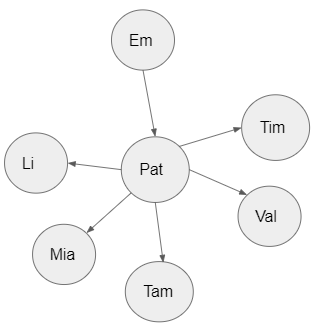Betweenness centrality can be applied to both a weighted and unweighted graph. For an unweighted graph, the shortest path between a pair of nodes is considered the betweenness centrality node. In terms of weighted graphs, the sum of weights between the edges that connect these vertices has to be the minimum. The nodes that are considered to have a high betweenness centrality are ones that produce the shortest paths (weighted and unweighted) to other vertices. In the case of the above figure, Pat becomes the betweenness centrality node as it is the quickest and shortest path for every pair of vertices.

The below is an expression of the betweenness centrality formula.

The betweenness centrality of a node equals to, how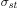is the total number of shortest paths from node s to node t and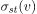is the number of those paths that pass through v. The number of paths that travels through v determines the betweenness centrality. There might be a couple of vertices that serve as a pathway for a pair of nodes but the total number of shortest paths through a given node of v in the formula above determines the node with the highest betweenness centrality or the most powerful in a given network.

Computing the shortest path of betweenness centrality of a node

The below formula is put forward on NetworkX in understanding the shortest path in relation to the betweenness centrality of a node.

The starting point is understanding the betweenness centrality of node v. It sums up the fraction of all-pairs shortest paths that travel through node v. In this case,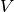is the set of nodes, while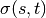is the number of shortest paths andlooks at the number of paths that travel through node v aside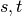Based on this expression, if s=t,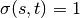, the number of nodes between s and t is expected to be one. Moving from node s to t, will be through a node. On the other hand, if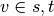,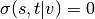. Considering node v is a member of s,t, the probability of some paths going through v other than s,t is zero. In this case, v is viewed as having a set membership to s and t, all shortest paths to v have to involve s,t.

Betweenness centrality measure for weighted graphs

In the case of weighted graphs, the respective weight of the edges are expected to be greater than zero. If weights of these edges fall below zero, there can be an issue of an infinite number of equal paths that travel through a pair of nodes.

Determining the betweenness centrality in Networkx

Networkx has a simple line of python codes to determine the betweenness centrality of nodes in a given graph. The below two lines of code was added to an existing weighted graph (G) that comprised 10 nodes.

b=nx.betweenness_centrality(G)
print(b)


The betweenness centrality score is usually a dictionary output. It determines the betweenness centrality of each node and in this case their respective weights are taken into consideration.

{1: 0.0, 2: 0.013888888888888888, 3: 0.041666666666666664, 4: 0.05555555555555555, 7: 0.15277777777777776, 10: 0.0, 5: 0.1111111111111111, 6: 0.1388888888888889, 8: 0.05555555555555555, 9: 0.0}



A print out of the graph with the above betweenness similarity dictionary looks like below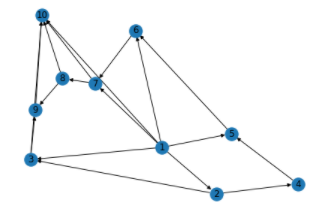By way of recap, the betweenness centrality algorithm captures centrality in a given network. The betweenness centrality of a given node is the number or sum of weights of the shortest paths that travel through the given vertex.